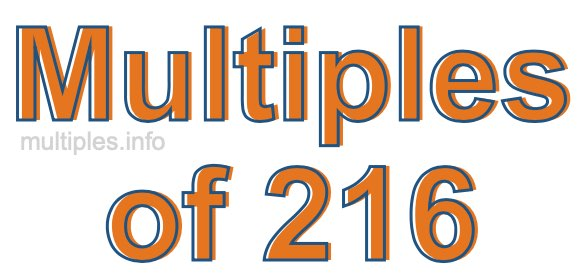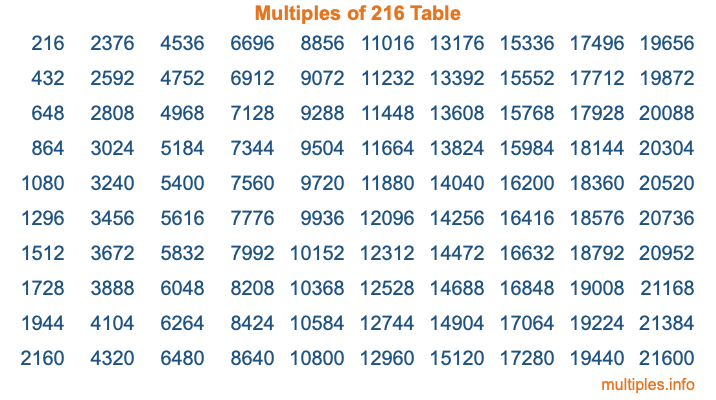Multiples of 216Welcome to the Multiples of 216 page. Here we will first teach you everything you will ever need to know about the multiples of 216, and then give you a study guide summary of everything we taught you to make sure you remember it all. Use this page to look up facts and learn information about the multiples of 216. This page will make you a multiples of two hundred sixteen expert!

Definition of Multiples of 216
Multiples of 216 are all the numbers that when divided by 216 equal an integer. Each of the multiples of 216 are called a multiple. A multiple of 216 is created by multiplying 216 by an integer.

Therefore, to create a list of multiples of 216, you start with 1 multiplied by 216, then 2 multiplied by 216, then 3 multiplied by 216, and so on for as long as you want. Thus, the list of the first five multiples of 216 is 216, 432, 648, 864, and 1080. To see a larger list of multiples of 216, see the printable image of Multiples of 216 further down on this page. We also have a category where you can choose any nth multiple of 216.

Multiples of 216 Checker
The Multiples of 216 Checker below checks to see if any number of your choice is a multiple of 216. In other words, it checks to see if there is any number (integer) that when multiplied by 216 will equal your number. To do that, we divide your number by 216. If the the quotient is an integer, then your number is a multiple of 216.

Is  a multiple of 216?

Least Common Multiple of 216 and ...
A Least Common Multiple (LCM) is the lowest multiple that two or more numbers have in common. This is also called the smallest common multiple or lowest common multiple and is useful to know when you are adding our subtracting fractions. Enter one or more numbers below (216 is already entered) to find the LCM.

Check out our LCM Calculator if you need more details about the Least Common Multiple or if you need the LCM for different numbers for adding and subtraction fractions.

nth Multiple of 216
As we stated above, 216 is the first multiple of 216, 432 is the second multiple of 216, 648 is the third multiple of 216, and so on. Enter a number below to find the nth multiple of 216.

th multiple of 216

Multiples of 216 vs Factors of 216
216 is a multiple of 216 and a factor of 216, but that is where the similarities end. All postive multiples of 216 are 216 or greater than 216. All positive factors of 216 are 216 or less than 216.

Below is the beginning list of multiples of 216 and the factors of 216 so you can compare:

Multiples of 216: 216, 432, 648, 864, 1080, etc.

Factors of 216: 1, 2, 3, 4, 6, 8, 9, 12, 18, 24, 27, 36, 54, 72, 108, 216

As you can see, the multiples of 216 are all the numbers that you can divide by 216 to get a whole number. The factors of 216, on the other hand, are all the whole numbers that you can multiply by another whole number to get 216.

It's also interesting to note that if a number (x) is a factor of 216, then 216 will also be a multiple of that number (x).

Multiples of 216 vs Divisors of 216
The divisors of 216 are all the integers that 216 can be divided by evenly. Below is a list of the divisors of 216.

Divisors of 216: 1, 2, 3, 4, 6, 8, 9, 12, 18, 24, 27, 36, 54, 72, 108, 216

The interesting thing to note here is that if you take any multiple of 216 and divide it by a divisor of 216, you will see that the quotient is an integer.

Multiples of 216 Table
Below is an image of the first 100 multiples of 216 in a table. The table is in chronological order, column by column. The first column has the first ten multiples of 216, the second column has the next ten multiples of 216, and so on.The Multiples of 216 Table is also referred to as the 216 Times Table or Times Table of 216. You are welcome to print out our table for your studies.

Negative Multiples of 216
Although not often discussed or needed in math, it is worth mentioning that you can make a list of negative multiples of 216 by multiplying 216 by -1, then by -2, then by -3, and so on, to get the following list of negative multiples of 216:

-216, -432, -648, -864, -1080, etc.

Multiples of 216 Summary
Below is a summary of important Multiples of 216 facts that we have discussed on this page. To retain the knowledge on this page, we recommend that you read through the summary and explain to yourself or a study partner why they hold true.

There are an infinite number of multiples of 216.

A multiple of 216 divided by 216 will equal a whole number.

216 divided by a factor of 216 equals a divisor of 216.

The nth multiple of 216 is n times 216.

The largest factor of 216 is equal to the first positive multiple of 216.

216 is a multiple of every factor of 216.

216 is a multiple of 216.

A multiple of 216 divided by a divisor of 216 equals an integer.

216 divided by a divisor of 216 equals a factor of 216.

Any integer times 216 will equal a multiple of 216.

Multiples of a Number
Here you can get the multiples of another number, all with the same attention to detail as we did for multiples of 216 on this page.

Multiples of
Multiples of 217
Did you find our page about multiples of two hundred sixteen educational? Do you want more knowledge? Check out the multiples of the next number on our list!